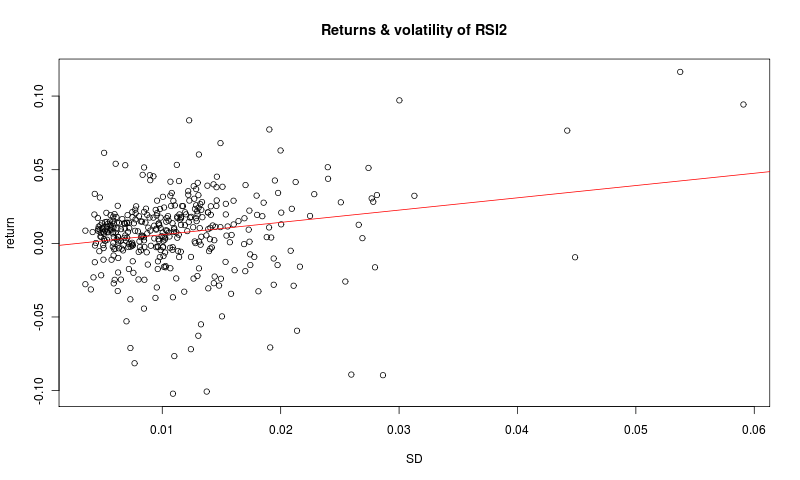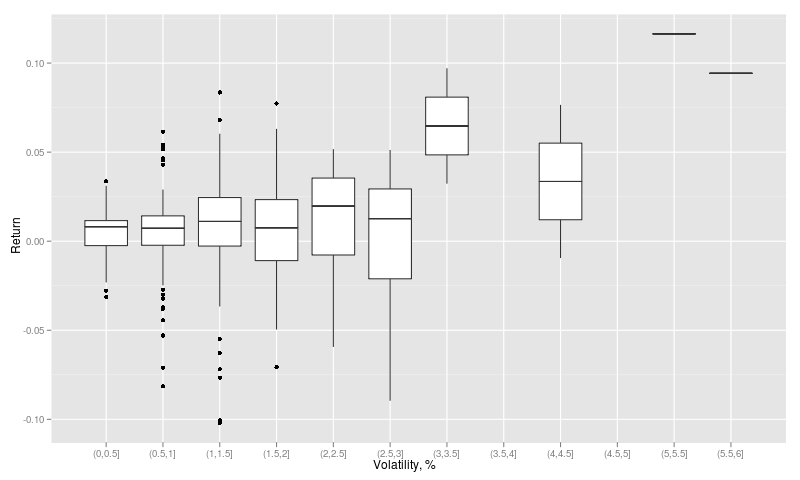# Mean reverting strategies and volatility

September 27, 2010
By

Want to share your content on R-bloggers? click here if you have a blog, or here if you don't.

Mean reverting strategies are beating on mean reversion of the prices. There are various flavors of mean reverting strategies, but as a proxy I chose RSI(2). You can find many entries on blogosphere about this strategy, but nowadays its popularity dried up.

What made me wondering is that there was an idea about correlation between return of such strategy and market volatility. That means, that during high volatility periods this strategy produces higher return and during low volatility -lower.

To test this idea, I built two strategies. RSI Simple goes long, if RSI(2) indicator is below 10 or it goes short, if RSi(2) is above 90. It closes the open position, then indicator is above/below 70/30 respectively.
RSI Garch follows the same rules as RSI Simple, except one additional rule. By using Garch model I forecast volatility for next day. If  volatility value is greater that 65 % of values during the year (252 days), then order is executed. By adding this filter I can catch most volatile days of the last year.

 RSI simple RSI Garch Profit factor 2.14 2.22 Winning % 0.69 0.7 Profit avg. 197 250 Loss avg. -207 -272 Sharpe 0.93 1.0278

The result of Garch filter are slightly better, but the question remains – is it worth of adding it?

Next thing I tried to look at correlation between volatility of the market and return. Actually, it was not so trivial to implement that. The problem is the duration of the investment, which is not fix in days (it can take between 1 to 19 days to close a position). So, I had two approaches- either measure volatility at the beginning of the investment or to fix max investment time (for example 5 days) and then measure volatility on the last day of investment. Despite the differences the results are similar and I will present former.
As you can see from the graph below there’s correlation between return of RSI(2) strategy and volatility of the last 20 days. However, I would attribute this correlation to the fact, that return (profit or loss) tend to be higher, then volatility is higher. This thought is supported by R^2, which was only 0.1.You can see return ranges against volatility ranges on the next graph. As in the first example above, I can’t see hard evidence of the correlation.Summary
Volatility filter can slightly improve return of RSI(2) strategy, but it is not significant. In the future I will run the same test on pairs trading strategy (another flavor of mean reverting strategies).

R-bloggers.com offers daily e-mail updates about R news and tutorials about learning R and many other topics. Click here if you're looking to post or find an R/data-science job.
Want to share your content on R-bloggers? click here if you have a blog, or here if you don't.

Tags: ,